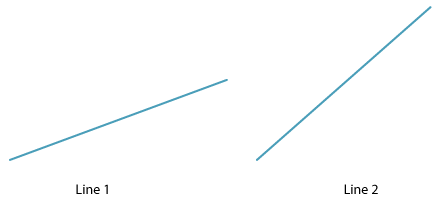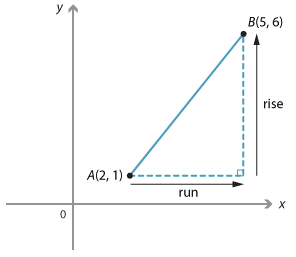Gradient is a measure of the steepness or slope of a line.Detailed description

We can see that Line 2 is steeper than Line 1.

There are several ways to measure steepness. In coordinate geometry the standard way to define the gradient of an interval AB is $$\dfrac{rise}{run}$$, where rise is the change in the y-values as you move from A to B, and run is the change in the x-values as you move from A to B. We will usually the pronumeral m for gradient.

#### Example 2Detailed description

Given the points A(2, 1) and B(5, 6):

\begin{align}\text{gradient of interval}\ AB &= \dfrac{rise}{run}\\ &=\dfrac{ 6− 1}{5 − 2}\\ &=\dfrac{5}{3}\end{align}

Notice that as you move from A to B along the interval the y-value increases as the x-value increases. The gradient is positive.